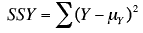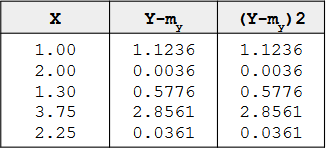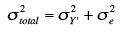# Statistics for Decision Makers - 14.02 - Regression - r squared

Title

14.02 - Regression - r squared
Author
Bernard Szlachta (NobleProg Ltd) bs@nobleprog.co.uk
Footer
www.NobleProg.co.uk
Subfooter

Training Courses Worldwide

## Dividing Variation。

Regression can divide the variation in Y into two parts:

• The variation of the predicted scores (Y)
• The variation in the errors of prediction (E)

### The variation of Y

• The sum of squares Y (SSY) or Total Sum of Squares (TSS)
• The sum of the squared deviations of Y from the mean of YSSY - the sum of squares Y and
Y   - an individual value of Y
μy is the mean of Y


## Example。

The mean of Y is 2.06 and SSY is the sum of the values in the third column and is equal to 4.597When computed using a sample, you should use the sample mean, M, in place of the population mean.

# Sum of the squared deviations from the mean。

SSY = SSY' + SSE


SSY can be partitioned into two parts:

1. The sum of squares predicted (SSY') or Explained Sum of Squares (ESS)
The sum of squares predicted is the sum of the squared deviations of the predicted scores from the mean predicted score (M')
$\sum _{i=1}^{n}(Y'-{M'})^{2}$2. The sum of squares error (SSE) or Residual Sum of Squares (RSS)
• The sum of squares error is the sum of the squared errors of prediction
$\sum _{i=1}^{n}(Y-Y')^{2}$## Proportion of variation explained。

SSY is the total variation
SSY' is the variation explained
SSE is the variation unexplained


Therefore, the proportion of variation explained can be computed as:

Proportion explained = SSY'/SSY


Similarly, the proportion not explained is:

Proportion not explained = SSE/SSY


## r2 and Pearson correlation。

There is an important relationship between the proportion of variation explained and Pearson's correlation:

r2 = SSY'/SSY = is the proportion of variation explained


Therefore,

• if r = 1, then the proportion of variation explained is 1
• if r = 0, then the proportion explained is 0;
• if r = 0.4, then the proportion of variation explained is 0.16

### Sum of Squares and Variances。

Variance is computed by dividing the variation (Sum of Squares) by N (for a population) or N-1 (for a sample). The relationships spelled out above in terms of variation also hold for variance.variance total = variance of prediction + errors of prediction


r2 is the proportion of
1. variance explained
2. variation explained

# Summary Table。

It is often convenient to summarize the partitioning of the data in a table.

• The degrees of freedom column (df) shows the degrees of freedom for each source of variation
• The degrees of freedom for the sum of squares explained is equal to the number of predictor variables
• This will always be 1 in simple regression
• The error degrees of freedom is equal to the total number of observations minus 2
• In this example, it is 5 - 2 = 3
• The total degrees of freedom is the total number of observations minus 1
Source Sum of Squares df Mean Square
Explained 1.806 1 1.806
Error 2.791 3 0.930
Total 4.597 4

## Understanding r2。

• AKA Coefficient of determination
• Goodness of fit of a model
• Measure of how well the regression line approximates the real data points
• In multiple regression it increases with number of predictors (see adjusted R2)

Example
r2 = 0.7

• 70% of the variation in the response variable can be explained by the explanatory variable
• 30% can be attributed to unknown, lurking variables or inherent variability

# Quiz

1

Compute the sum of squares Y.

 Y
2
9
11
13
15


100

To compute SSY, first compute the deviation scores (y) by subtracting the mean (10) from each number. Then square these values and add them together: (-8)2 + (-1)2 + 12 + 32 + 52 equals 100

2

If SSY is 25.5 and SSY' is 18.3, what is SSE?

7.2

SSY is SSY' + SSE; SSE is SSY - SSY' 25.5 - 18.3 equals 7.2

3

The larger ________ is, the larger the proportion of variation explained is.

 SSY SSY' SSE Y

False

Proportion of variation explained is SSY'/SSY, so as SSY' increases, so does the proportion of variation explained.

4

The proportion of variation explained is 0.3. If SSY is 20, what is SSY'?

6

Proportion explained is SSY'/SSY; SSY' is (.3)(20) equals to 6

5

If r is .84, what proportion of variation is explained?

0.71

r2 is the proportion of variation explained. (.84)2 is .71

6

A company created a model of how advertisement duration impacts the effectiveness of online video advertising. A simple linear regression model was used. The coefficient of determination was 0.2 How should the manager react?

 discard the model as it is invalid . The value of 0.2 is far too low to be significant look for variables more relevant to the effectiveness of advertising repeat the same experiment until r2 is higher than 0.5